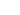# VLOOKUP Function

This article explains how to use VLOOKUP functions

# Sample Usage

``VLOOKUP(QA, DA, 1, 0)``
``VLOOKUP(135, DB, 2, 1)``

# Syntax

``VLOOKUP (value, datasheet, col_index, [range_lookup])``
• `value` - The value to look for in the first column of a datasheet.
• `datasheet` - The table from which to retrieve a value.
• `col_index` - The column in the datasheet from which to retrieve a value.
• `range_lookup` - [optional] 0 = exact match (default). 1 = approximate match.
• `range_lookup_higher_bound` - [optional] 0 = return lower value (default). 1 = return higher value
• Instead of a table range in a spreadsheet program (e.g. `A1:B22`), the value of the datasheet needs to be just the reference to that datasheet (eg. `DA`).